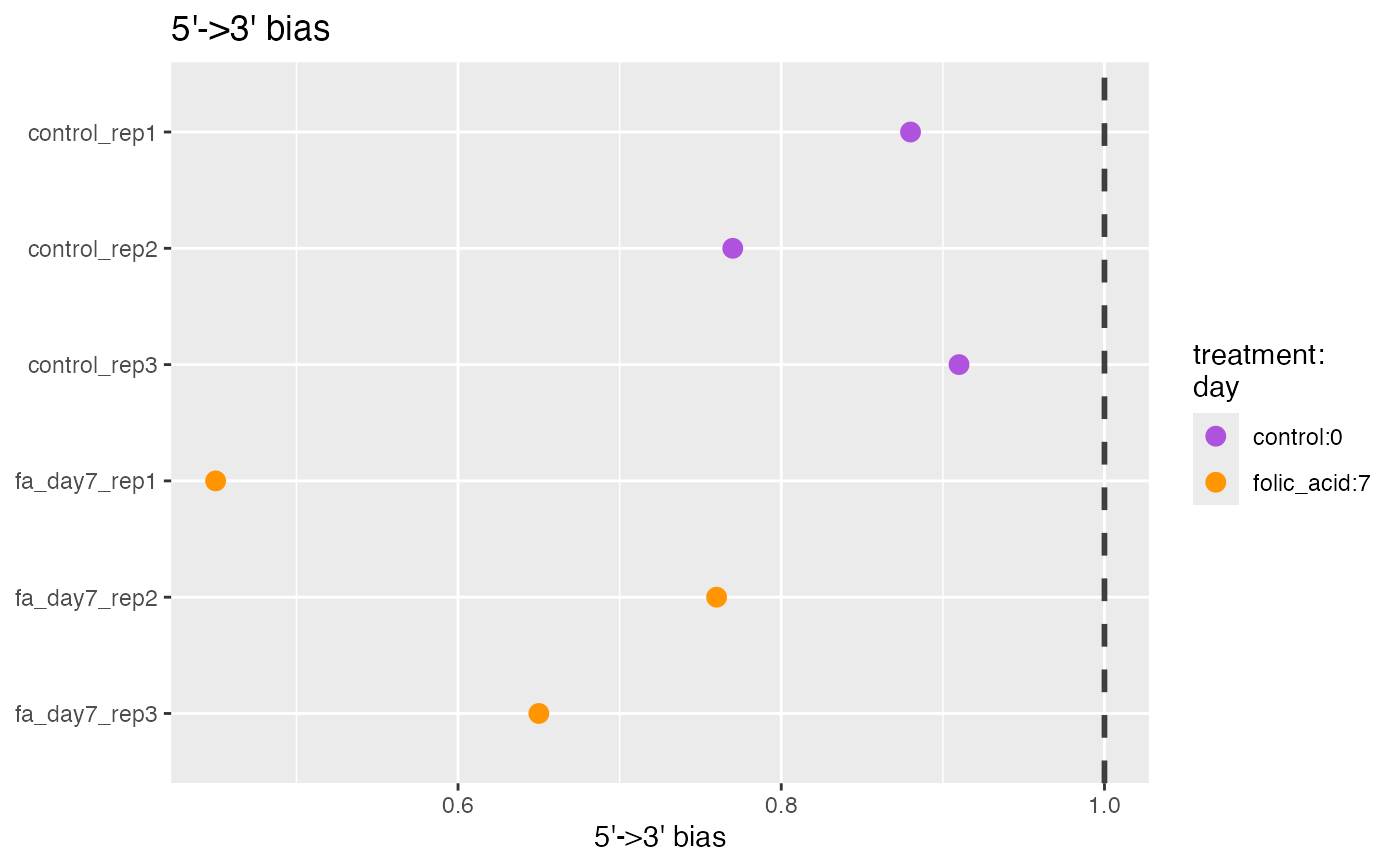RNA-seq data can have specific biases at either the 5’ or 3’ end of sequenced fragments.

## Usage

plot5Prime3PrimeBias(object, ...)

# S4 method for bcbioRNASeq
plot5Prime3PrimeBias(
object,
interestingGroups = NULL,
labels = list(title = "5'->3' bias", subtitle = NULL, sampleAxis = NULL, metricAxis =
"5'->3' bias"),
flip = getOption(x = "acid.flip", default = TRUE)
)

## Arguments

object

Object.

interestingGroups

character. Groups of interest to use for visualization. Corresponds to factors describing the columns of the object.

labels

list. ggplot2 labels. See ggplot2::labs() for details.

flip

logical(1). Flip x and y axes. Recommended for plots containing many samples.

...

## Value

ggplot.

## Details

It is common to see a small amount of bias, especially if polyA enrichment was performed, or if there is any sample degradation. If a large amount of bias is observed here, be sure to analyze the samples with a Bioanalyzer and check the RIN scores.

5' (3') bias is generally calculated as the median of the following ratio:

[mean expression of 5' (3')] /
[mean expression of whole transcript]

For example:

• Mean expression for 5' (3') is calculated as mean coverage of first (last) 100 bases.

• Mean expression of transcript is the mean coverage of all bases in that transcript.

• Median is calculated for the representative set of 1000 transcripts.

## Note

Updated 2022-05-09.

## Author

Michael Steinbaugh

## Examples

data(bcb)

## bcbioRNASeq ====
plot5Prime3PrimeBias(bcb)